Share

# RD Sharma solutions for Class 8 Maths chapter 17 - Understanding Shapes-III (Special Types of Quadrilaterals)

#### RD Sharma Mathematics Class 8 by R D Sharma (2019-2020 Session)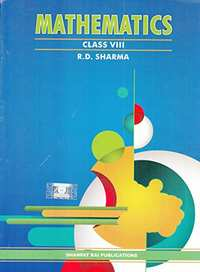## Chapter 17: Understanding Shapes-III (Special Types of Quadrilaterals)

Ex. 17.1Ex. 17.2Ex. 17.3

#### RD Sharma solutions for Class 8 Maths Chapter 17 Exercise 17.1 [Pages 9 - 12]

Ex. 17.1 | Q 1 | Page 9

Given below is a parallelogram ABCD. Complete each statement along with the definition or property used.
(ii) ∠DCB =
(iii) OC =
(iv) ∠DAB + ∠CDA =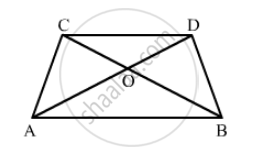Ex. 17.1 | Q 2.1 | Page 9

The following figure is parallelogram. Find the degree values of the unknowns xyz.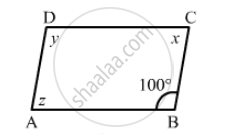Ex. 17.1 | Q 2.2 | Page 9

The following figure is parallelogram. Find the degree values of the unknown xyz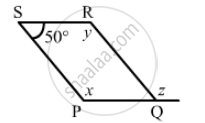Ex. 17.1 | Q 2.3 | Page 9

The following figure is parallelogram. Find the degree values of the unknown xyz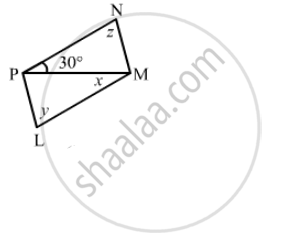Ex. 17.1 | Q 2.4 | Page 9

The following figure is parallelogram. Find the degree values of the unknown xyz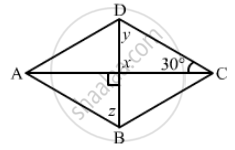Ex. 17.1 | Q 2.5 | Page 9

The following figure is parallelogram. Find the degree values of the unknown xyz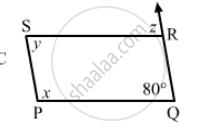Ex. 17.1 | Q 2.6 | Page 9

The following figure is parallelogram. Find the degree value of the unknown xyz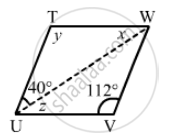Ex. 17.1 | Q 3.1 | Page 10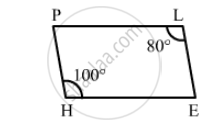Ex. 17.1 | Q 3.2 | Page 10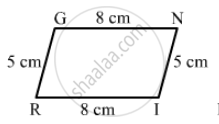Ex. 17.1 | Q 3.3 | Page 10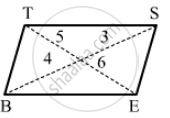Ex. 17.1 | Q 4 | Page 10

In the adjacent figure HOPE is a parallelogram. Find the angle measures x,y and z. State the geometrical truths you use to find them.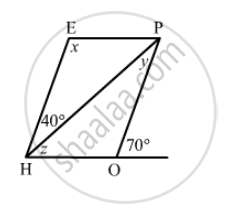Ex. 17.1 | Q 5.1 | Page 10

In the following figure GUNS and RUNS are  parallelogram. Find x and y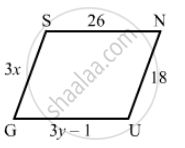Ex. 17.1 | Q 5.2 | Page 10

In the following figure GUNS and RUNS are  parallelogram. Find x and y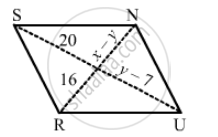Ex. 17.1 | Q 6 | Page 10

In the following figure RISK and CLUE are parallelograms. Find the measure of x.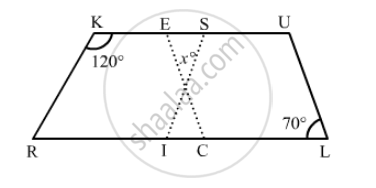Ex. 17.1 | Q 7 | Page 10

Two opposite angles of a parallelogram are (3x − 2)° and (50 − x)°. Find the measure of each angle of the parallelogram.

Ex. 17.1 | Q 8 | Page 10

If an angle of a parallelogram is two-third of its adjacent angle, find the angles of the parallelogram.

Ex. 17.1 | Q 9 | Page 10

The measure of one angle of a parallelogram is 70°. What are the measures of the remaining angles?

Ex. 17.1 | Q 10 | Page 10

Two adjacent angles of a parallelogram are as 1 : 2. Find the measures of all the angles of the parallelogram.

Ex. 17.1 | Q 11 | Page 10

In a parallelogram ABCD, ∠D = 135°, determine the measure of ∠A and ∠B.

Ex. 17.1 | Q 12 | Page 11

ABCD is a parallelogram in which ∠A = 70°. Compute ∠BC and D.

Ex. 17.1 | Q 13 | Page 11

The sum of two opposite angles of a parallelogram is 130°. Find all the angles of the parallelogram.

Ex. 17.1 | Q 14 | Page 11

All the angles of a quadrilateral are equal to each other. Find the measure of each. Is the quadrilateral a parallelogram? What special type of parallelogram is it?

Ex. 17.1 | Q 15 | Page 11

Two adjacent sides of a parallelogram are 4 cm and 3 cm respectively. Find its perimeter.

Ex. 17.1 | Q 16 | Page 11

The perimeter of a parallelogram is 150 cm. One of its sides is greater than the other by 25 cm. Find the length of the sides of the parallelogram.

Ex. 17.1 | Q 17 | Page 11

The shorter side of a parallelogram is 4.8 cm and the longer side is half as much again as the shorter side. Find the perimeter of the parallelogram.

Ex. 17.1 | Q 18 | Page 11

Two adjacent angles of a parallelogram are (3x − 4)° and (3x + 10)°. Find the angles of the parallelogram.

Ex. 17.1 | Q 19 | Page 11

In a parallelogram ABCD, the diagonals bisect each other at O. If ∠ABC = 30°, ∠BDC = 10° and ∠CAB = 70°. Find:
DAB, ∠ADC, ∠BCD, ∠AOD, ∠DOC, ∠BOC, ∠AOB, ∠ACD, ∠CAB, ∠ADB, ∠ACB, ∠DBC and ∠DBA.

Ex. 17.1 | Q 20 | Page 11

Find the angles marked with a question mark shown in Fig. 17.27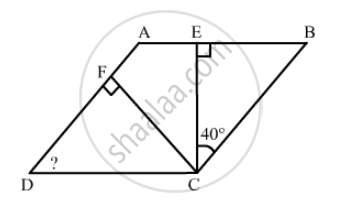Ex. 17.1 | Q 21 | Page 11

The angle between the altitudes of a parallelogram, through the same vertex of an obtuse angle of the parallelogram is 60°. Find the angles of the parallelogram.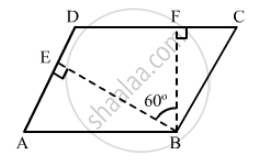Ex. 17.1 | Q 22 | Page 11

In the following figure, ABCD and AEFG are parallelograms. If ∠C = 55°, what is the measure of ∠F?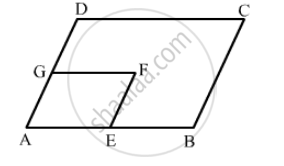Ex. 17.1 | Q 23 | Page 11

In the following figure, BDEF and DCEF are each a parallelogram. Is it true that BD = DC? Why or why not?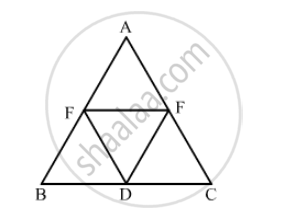Ex. 17.1 | Q 24 | Page 12

In Fig. 17.29, suppose it is known that DE = DF. Then, is ΔABC isosceles? Why or why not?

Ex. 17.1 | Q 25 | Page 12

Diagonals of parallelogram ABCD intersect at O as shown in the following fegure. XY contains O, and XY are points on opposite sides of the parallelogram. Give reasons for each of the following:
(i) OB = OD
(ii) ∠OBY = ∠ODX
(iii) ∠BOY = ∠DOX
(iv) ∆BOY ≅ ∆DOX
Now, state if XY is bisected at O.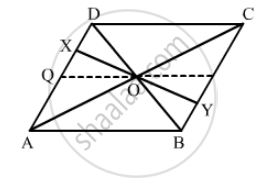Ex. 17.1 | Q 26 | Page 12

In the following  Figure  ABCD is a  arallelogram, CE bisects ∠C and AF bisects ∠A. In each of the following, if the statement is true, give a reason for the same: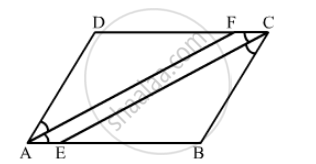(i) ∠A = ∠C
(ii) $\angle FAB = \frac{1}{2}\angle A$

(iii) $\angle DCE = \frac{1}{2}\angle C$

(iv) $\angle CEB = \angle FAB$

(v) CE || AF

Ex. 17.1 | Q 27 | Page 12

Diagonals of a parallelogram ABCD intersect at OAL and CM are drawn perpendiculars to BD such that L and M lie on BD. Is AL = CM? Why or why not?

Ex. 17.1 | Q 28 | Page 12

Points E and F lie on diagonal AC of a parallelogram ABCD such that AE = CF. What type of quadrilateral is BFDE?

Ex. 17.1 | Q 29 | Page 12

In a parallelogram ABCDAB = 10 cm, AD = 6 cm. The bisector of ∠A meets DC in EAEand BC produced meet at F. Find te length CF

#### RD Sharma solutions for Class 8 Maths Chapter 17 Exercise 17.2 [Pages 16 - 17]

Ex. 17.2 | Q 1.01 | Page 16

Which of the following statement is true for a rhombus?

It has two pairs of parallel sides.

Ex. 17.2 | Q 1.02 | Page 16

Which of the following statement is true for a rhombus?

It has two pairs of equal sides.

Ex. 17.2 | Q 1.03 | Page 16

Which of the following statement is true for a rhombus?

It has only two pairs of equal sides.

Ex. 17.2 | Q 1.04 | Page 16

Which of the following statement is true for a rhombus?

Two of its angles are at right angles

Ex. 17.2 | Q 1.05 | Page 16

Which of the following statement is true for a rhombus?

Its diagonals bisect each other at right angles.

Ex. 17.2 | Q 1.06 | Page 16

Which of the following statement is true for a rhombus?

Its diagonals are equal and perpendicular.

Ex. 17.2 | Q 1.07 | Page 16

Which of the following statement is true for a rhombus?

It has all its sides of equal lengths.

Ex. 17.2 | Q 1.08 | Page 16

Which of the following statement is true for a rhombus?

It is a parallelogram.

Ex. 17.2 | Q 1.09 | Page 16

Which of the following statement is true for a rhombus?

Ex. 17.2 | Q 1.1 | Page 16

Which of the following statement is true for a rhombus?

It can be a square.

Ex. 17.2 | Q 1.11 | Page 16

Which of the following statement is true for a rhombus?

It is a square.

Ex. 17.2 | Q 2.1 | Page 17

Fill in the blank, in the following, so as to make the statement true:

A rhombus is a parallelogram in which ......

Ex. 17.2 | Q 2.2 | Page 17

Fill in the blank, inthe following, so as to make the statement true:

A square is a rhombus in which .....

Ex. 17.2 | Q 2.3 | Page 17

Fill in the blank, inthe following, so as to make the statement true:

A rhombus has all its sides of ...... length.

Ex. 17.2 | Q 2.4 | Page 17

Fill in the blank, in the following, so as to make the statement true:

The diagonals of a rhombus ...... each other at ...... angles.

Ex. 17.2 | Q 2.5 | Page 17

Fill in the blank, in each of the following, so as to make the statement true:

If the diagonals of a parallelogram bisect each other at right angles, then it is a ......

Ex. 17.2 | Q 3 | Page 17

The diagonals of a parallelogram are not perpendicular. Is it a rhombus? Why or why not?

Ex. 17.2 | Q 4 | Page 17

Ex. 17.2 | Q 5 | Page 17

ABCD is a rhombus. If ∠ACB = 40°, find ∠ADB.

Ex. 17.2 | Q 6 | Page 17

If the diagonals of a rhombus are 12 cm and 16cm, find the length of each side.

Ex. 17.2 | Q 7 | Page 17

Construct a rhombus whose diagonals are of length 10 cm and 6 cm.

Ex. 17.2 | Q 8 | Page 17

Draw a rhombus, having each side of length 3.5 cm and one of the angles as 40°.

Ex. 17.2 | Q 9 | Page 17

One side of a rhombus is of length 4 cm and the length of an altitude is 3.2 cm. Draw the rhombus.

Ex. 17.2 | Q 10 | Page 17

Draw a rhombus ABCD, if AB = 6 cm and AC = 5 cm.

Ex. 17.2 | Q 11 | Page 17

ABCD is a rhombus and its diagonals intersect at O.
(i) Is ∆BOC ≅ ∆DOC? State the congruence condition used?
(ii) Also state, if ∠BCO = ∠DCO.

Ex. 17.2 | Q 12 | Page 17

Show that each diagonal of a rhombus bisects the angle through which it passes.

Ex. 17.2 | Q 13 | Page 17

ABCD is a rhombus whose diagonals intersect at O. If  AB = 10 cm, diagonal BD = 16 cm, find the length of diagonal AC.

Ex. 17.2 | Q 14 | Page 17

The diagonals of a quadrilateral are of lengths 6 cm and 8 cm. If the diagonals bisect each other at right angles, what is the length of each side of the quadrilateral?

#### RD Sharma solutions for Class 8 Maths Chapter 17 Exercise 17.3, 17.2 [Pages 22 - 23]

Ex. 17.3 | Q 1.01 | Page 22

Which of the following statement is true for a rectangle?

It has two pairs of equal sides.

Ex. 17.3 | Q 1.02 | Page 22

Which of the following statement is true for a rectangle?

It has all its sides of equal length.

Ex. 17.3 | Q 1.03 | Page 22

Which of the following statement is true for a rectangle?

Its diagonals are equal.

Ex. 17.3 | Q 1.04 | Page 22

Which of the following statement is true for a rectangle?

Its diagonals bisect each other.

Ex. 17.3 | Q 1.05 | Page 22

Which of the following statement is true for a rectangle?

Its diagonals are perpendicular.

Ex. 17.3 | Q 1.06 | Page 22

Which of the following statement is true for a rectangle?

Its diagonals are perpendicular and bisect each other.

Ex. 17.3 | Q 1.07 | Page 22

Which of the following statement is true for a rectangle?

Its diagonals are equal and bisect each other.

Ex. 17.3 | Q 1.08 | Page 22

Which of the following statement is true for a rectangle?

Its diagonals are equal and perpendicular, and bisect each other.

Ex. 17.3 | Q 1.09 | Page 22

Which of the following statement is  true for a rectangle?

All rectangles are squares.

Ex. 17.3 | Q 1.1 | Page 22

Which of the following statement is true for a rectangle?

All rhombuses are parallelograms.

Ex. 17.3 | Q 1.11 | Page 22

Which of the following statement is true for a rectangle?

All squares are rhombuses and also rectangles.

Ex. 17.3 | Q 1.12 | Page 22

Which of the following statementis true for a rectangle?

All squares are not parallelograms.

Ex. 17.3 | Q 2.1 | Page 22

Which of the following statement is true for a square?

It is a rectangle.

Ex. 17.3 | Q 2.2 | Page 22

Which of the following statement are true for a square?

It has all its sides of equal length.

Ex. 17.3 | Q 2.3 | Page 22

Which of the following statement  true for a square?

Its diagonals bisect each other at right angle.

Ex. 17.3 | Q 2.4 | Page 22

Which of the following statement  true for a square?

Its diagonals are equal to its sides.

Ex. 17.3 | Q 3.1 | Page 23

Fill in the blank in the following, so as to make the statement true:

A rectangle is a parallelogram in which .....

Ex. 17.3 | Q 3.2 | Page 23

Fill in the blank in  the following, so as to make the statement true:

A square is a rhombus in which .....

Ex. 17.3 | Q 3.3 | Page 23

Fill in the blank of the following, so as to make the statement true:

A square is a rectangle in which .....

Ex. 17.3 | Q 4 | Page 23

A window frame has one diagonal longer than the other. Is the window frame a rectangle? Why or why not?

Ex. 17.3 | Q 5 | Page 23

In a rectangle ABCD, prove that ∆ACB ≅ ∆CAD.

Ex. 17.3 | Q 6 | Page 23

The sides of a rectangle are in the ratio 2 : 3, and its perimeter is 20 cm. Draw the rectangle.

Ex. 17.3 | Q 7 | Page 23

The sides of a rectangle are in the ratio 4 : 5. Find its sides if the perimeter is 90 cm.

Ex. 17.3 | Q 8 | Page 23

Find the length of the diagonal of a rectangle whose sides are 12 cm and 5 cm.

Ex. 17.3 | Q 9 | Page 23

Draw a rectangle whose one side measures 8 cm and the length of each of whose diagonals is 10 cm.

Ex. 17.3 | Q 10 | Page 23

Draw a square whose each side measures 4.8 cm.

Ex. 17.2 | Q 11.1 | Page 23

Identify all the quadrilaterals that have:

Four sides of equal length

Ex. 17.3 | Q 11.2 | Page 23

Identify all the quadrilaterals that have:

Four right angles

Ex. 17.3 | Q 12.1 | Page 23

Explain how a square is a quadrilateral?

Ex. 17.3 | Q 12.2 | Page 23

Explain how a square is  a parallelogram?

Ex. 17.3 | Q 12.3 | Page 23

Explain how a square is a rhombus?

Ex. 17.3 | Q 12.4 | Page 23

Explain how a square is a rectangle?

Ex. 17.3 | Q 13.1 | Page 23

Name the quadrilaterals whose diagonals bisect each other

Ex. 17.3 | Q 13.2 | Page 23

Name the quadrilaterals whose diagonal are perpendicular bisector of each other

Ex. 17.3 | Q 13.3 | Page 23

Name the quadrilaterals whose diagonals are equal.

Ex. 17.3 | Q 14 | Page 23

ABC is a right-angled trianle and O is the mid-point of the side opposite to the right angle. Explain why O is equidistant from AB and C.

Ex. 17.3 | Q 15 | Page 23

A mason has made a concrete slab. He needs it to be rectangular. In what different ways can he make sure that it is rectangular?

## Chapter 17: Understanding Shapes-III (Special Types of Quadrilaterals)

Ex. 17.1Ex. 17.2Ex. 17.3

#### RD Sharma Mathematics Class 8 by R D Sharma (2019-2020 Session)## RD Sharma solutions for Class 8 Mathematics chapter 17 - Understanding Shapes-III (Special Types of Quadrilaterals)

RD Sharma solutions for Class 8 Maths chapter 17 (Understanding Shapes-III (Special Types of Quadrilaterals)) include all questions with solution and detail explanation. This will clear students doubts about any question and improve application skills while preparing for board exams. The detailed, step-by-step solutions will help you understand the concepts better and clear your confusions, if any. Shaalaa.com has the CBSE Mathematics for Class 8 by R D Sharma (2019-2020 Session) solutions in a manner that help students grasp basic concepts better and faster.

Further, we at Shaalaa.com provide such solutions so that students can prepare for written exams. RD Sharma textbook solutions can be a core help for self-study and acts as a perfect self-help guidance for students.

Concepts covered in Class 8 Mathematics chapter 17 Understanding Shapes-III (Special Types of Quadrilaterals) are Regular and Irregular Polygons, Angle Sum Property, Sum of the Measures of the Exterior Angles of a Polygon, Concept of Trapezium, Concept of Kite, Elements of a Parallelogram, Diagonals of a Parallelogram, Concept of Rhombus, Concept of a Rectangle, Classification of Polygons, Concept of Diagonals, Convex and Concave Polygons, Introduction of Quadrilaterals, Concept of a Square, Concept of Parallelogram.

Using RD Sharma Class 8 solutions Understanding Shapes-III (Special Types of Quadrilaterals) exercise by students are an easy way to prepare for the exams, as they involve solutions arranged chapter-wise also page wise. The questions involved in RD Sharma Solutions are important questions that can be asked in the final exam. Maximum students of CBSE Class 8 prefer RD Sharma Textbook Solutions to score more in exam.

Get the free view of chapter 17 Understanding Shapes-III (Special Types of Quadrilaterals) Class 8 extra questions for Maths and can use Shaalaa.com to keep it handy for your exam preparation

S